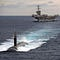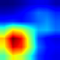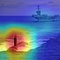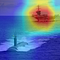# CNN Explainer - Interpreting Convolutional Neural Networks (2/N)

## Visualizing Gradient Weighted Class Activations with GradCAM

In today’s article, we are going to visualize gradient weighted class activations. It may sound confusing at first, but at the end of this article, you will be able to ‘ask’ Convolutional Neural Networks (CNNs) for visual explanations of their predictions. In other words, you will be able to highlight image regions responsible for predicting a given class.

This is the second part of the CNN Explainer series. If you haven’t checked the first part yet, feel free to do it now.

# Why Interpretability Matters?

Let’s consider the following input image:(source: https://www.activistpost.com/2016/11/us-navy-plans-release-20000-tons-explosives-heavy-metals-pacific-ocean.html)

A simple CNN classifier would probably predict that this is a submarine, and it wasn’t different for our Resnet18 pretrained on imagenet.

It’s a submarine with 95% confidence and an aircraft carrier with 5%.

`# submarine ~95%, aircraft carrier ~5%torch.return_types.topk(values=tensor([[0.9504, 0.0472]], grad_fn=<TopkBackward>),indices=tensor([[833, 403]]))`

It’s hard to argue with that result, because we can see that the above image contains both the submarine and an aircraft carrier, but that’s it - CNN’s don’t give us more information out of box.

But what if we are more inquisitive and need more information?

Trying to localize submarine and aircraft carrier might sounds like a good start, that should bring us closer to seeing the ‘full picture’.

# GradCAM

One of the approaches to highlight class activations (thus showing us class localizations) is GradCAM, that was developed in 2016 by Selvaraju et. al..

The idea behind GradCAM is quite simple yet very powerful, but before we go into the details, we need to review how feedforward neural networks work to fully understand it.

## Simplified Feedforward Neural Network Flow

Firstly, we pass an input through the network’s layers and get some output. It’s called the forward pass.

`x -> layer_0 -> {...} -> layer_n -> y`

Outputs of the intermediate layers given some input can be called activations. Layers are interspersed with non-linear activation functions, hence the name.

You can think of activations as a layer’s current perception of an input.

Then, using the loss function, we calculate the loss between the output and the ground truth.

Finally, having the loss value, we can do the backpropagation on the network, and as the name suggests, propagate the error backward. However, we don’t want to change all weights in the same way.

During the backward pass, in order to improve the network, some weights should be increased, some should be decreased and some of them should remain the same. Such rates of weight changes are called gradients.

You can think of gradients as a way (direction) to improve the layer’s perception of an input.

With the above flow, a neural network can gradually improve over time.

Now, knowing what are gradients and activations, we can proceed to the GradCAM implementation.

## GradCAM Implementation

Feel free to check the corresponding codebase:

We start by selecting our target layer. The rule of thumb here is to pick the last convolutional layer, the one that’s just before the classifier. The reasoning behind this is to pick the layer that has all information necessary to come up with the final prediction.

`gradcam = GradCam(model=model, target_layer=model.layer4, target_layer_names=[“1”])`

Under the hood, gradcam creates a layer_extractor object that acts like the man-in-the-middle and intercepts the information at the given target layer. More specifically, it collects activations and gradients.

The next step would be to select the target class, i.e class that we would like to localize. In our case, it’s a submarine, class 833. The full list of classes is available there.

Then we can feed the gradcam object with the input image and the target class.

`cam_heatmap = gradcam(preprocessed_image, target_class)`

During the forward pass, gradcam stores activations at the target layer.

Now comes ‘the magic’, where we have to query the network for our target class. In order to do it, we create a vector of size 1000 (length of the output, number of classes) that has all zeroes except for the index of our target class which gets one.

`one_hot = np.zeros((1, output.size()[-1]), dtype=np.float32)one_hot[target_class] = 1`

Then we multiple such a vector with the output vector that we initially received, and do the backward pass with the result.

`one_hot = torch.from_numpy(one_hot).requires_grad_(True)one_hot = torch.sum(one_hot * output)self.target_layer.zero_grad()self.model.zero_grad()one_hot.backward(retain_graph=True)`

Setting loss to 1 for a target class, we are essentially giving the network an information that it did horribly wrong for that class and it should significantly improve ‘responsible’ weights.

The idea behind heavily punishing the network for the target class is that it’s going to try to ‘repair itself’ via backpropagation, and by doing that, it’s going to show us which weights ‘need to be repaired’, thus exposing the localizations we are looking for.

After the backward pass we can grab the gradients from the backward pass

`gradients = self.layer_extractor.get_gradients()[-1].cpu().data.numpy()gradients = np.mean(gradients, axis=(2, 3))[0, :]`

and activations from the forward pass

`activations = activations[-1]activations = activations().data.numpy()[0, :]`

Then we can multiple corresponding activations and gradients values to get a matrix that stores an information about the ‘repaired’ target class.

`cam = np.zeros(activations.shape[1:], dtype=np.float32) for i, gradient in enumerate(gradients):     cam += gradient * activations[i, :, :]`

Such a matrix as a normalized grayscale jet heatmap looks like this:(source: author)

# Results

Finally, we can overlay it over the input image:(source: author)

Isn’t this exactly what we were looking for?

GradCAM showed us the area responsible for predicting the target class which was the submarine.

Let’s try the same with the aircraft carrier target class.(source: author)

GradCAM accurately highlighted both the submarine and the aircraft carrier proving its capabilities as a CNN visual explanation method.

With the target class localizations:

1. We can be more confident about CNN’s predictions.
2. We can derive insights and have a broader understanding of the scene.

In this particular case, we could hypothesize that given the locations of the submarine and the aircraft carrier, and the wake of water between them, the aircraft carrier is probably following the submarine.

# What’s Next?

Visualizing gradient weighted class activations with GradCAM is a great way to boost the understandability of CNN’s predictions. However, there is still more to come in the CNN Explainer series - and the next part is already out!

And don’t forget to 👏 if you enjoyed this article 🙂.

## More from Greg Surma

https://gsurma.github.io